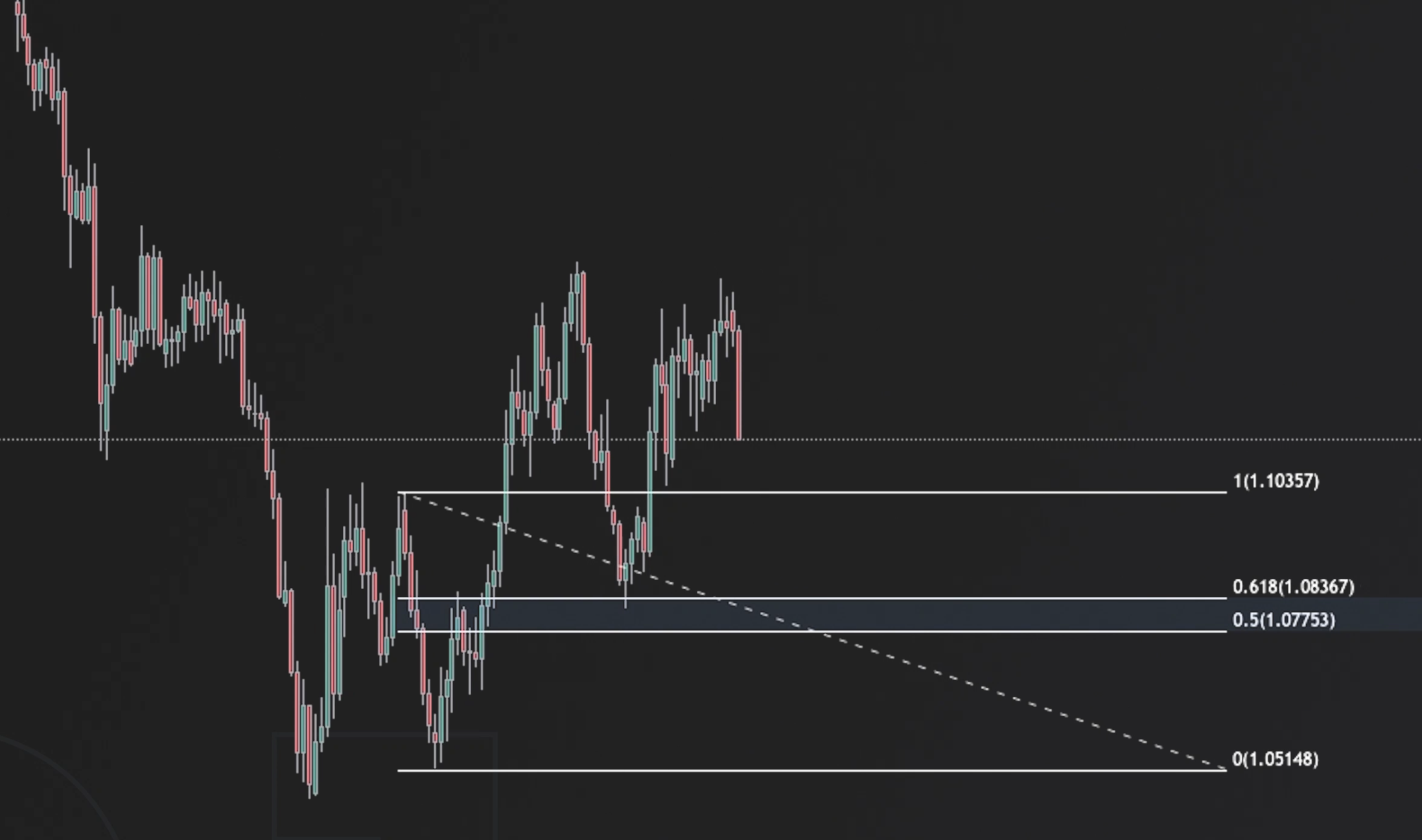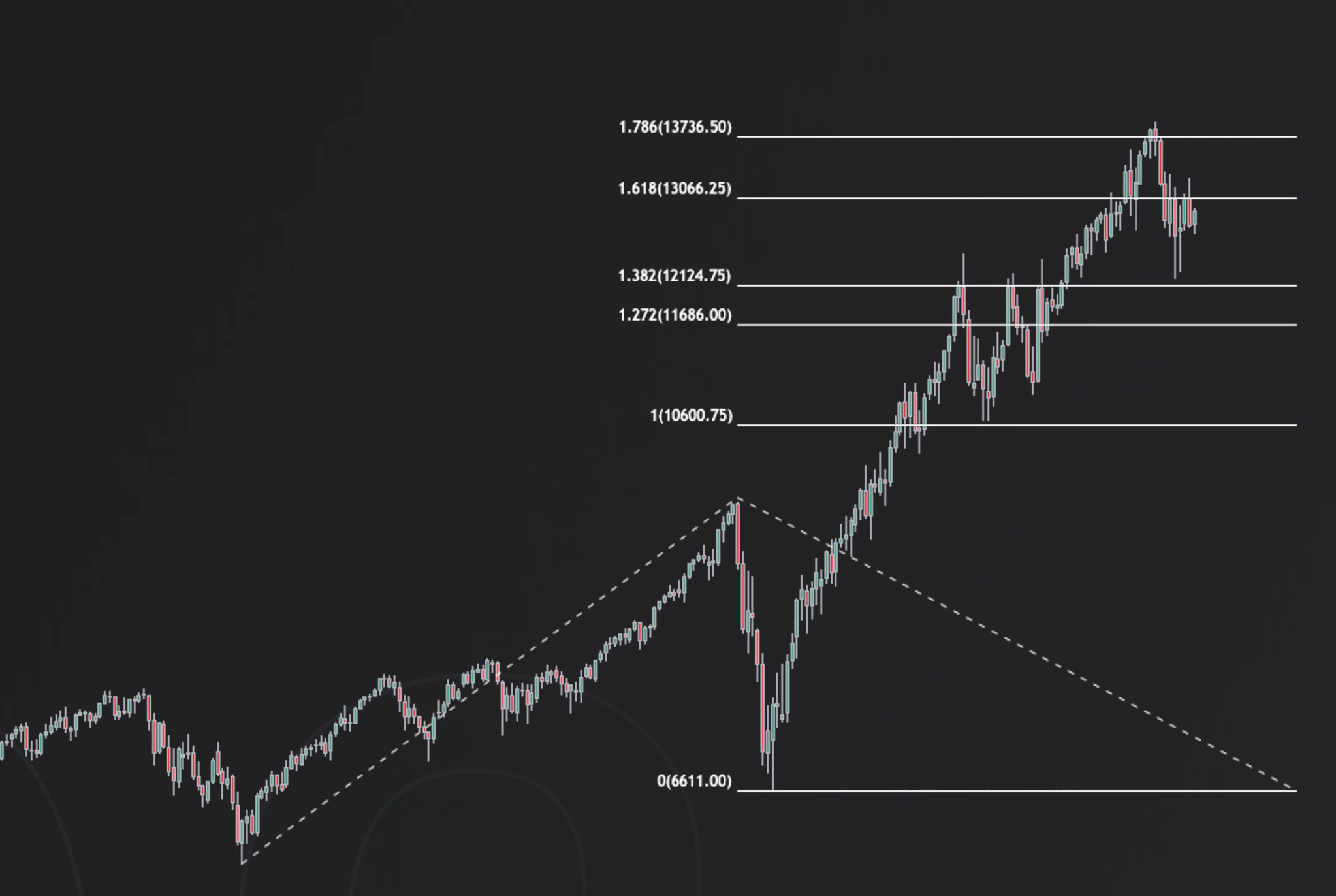In this lesson, we will have a look at Fibonacci retracement numbers and the meaning behind them.

## Fibonacci retracements

The Fibonacci retracements are one of the most popular tools in technical analysis. They came up from the Fibonacci sequence of numbers. These are 0, 1, 1, 2, 3, 5, 8, 13, 21, 34, 55, 89, 144… If we divide two numbers next to each other from number three and above, we will gain a certain ratio we can use from Fibonacci retracement levels. Fibonacci retracement levels can then be used as horizontal support and resistance areas. And they are created by using a Fibonacci retracement tool which is available with pretty much every trading software and plotting it from key swing highs and swing lows. Once we plot those, we will see 23.6, 38.2, .50, 61.8, and 100% retracement numbers.

Why do these numbers work?

There is no magic behind that; it is simply because dozens of traders watch them, so we tend to see a reaction once the price reaches them. The most important ones are 38.2, 50 and 61.8% retracement levels. The 61.8% retracement is also called the golden ratio, and it is the most important one. A 50% retracement is not technically a Fibonacci number, but it is used because it works.As we can see on this chart, this is a daily EURUSD chart where we plotted a Fibonacci retracement tool on key swing highs and swing lows.  Once the price starts its retracement, we could find a buying opportunity between 61,8 and 50% retracement. Another way of using Fibonacci numbers is with a Fibonacci extension.

### Fibonacci extension

This Fibonacci extension can predict future support and resistance levels in trending markets. This extension tool is pulled from major swing moves, and it is beneficial with instruments in price discovery. In other words, instruments that are beyond their previous all-time high.If we take this NASDAQ chart, we can see that the Fibonacci extension was pulled from the whole 2020 March crash. It could show us important levels of support and resistance in play after NASDAQ broke its all-time high. In conclusion, Fibonacci retracement and extension can be useful, but it is not the holy grail in trading. These levels oftentimes get front-run or broken through, so practising good risk management is always the key.